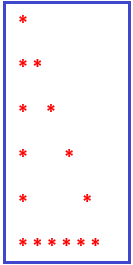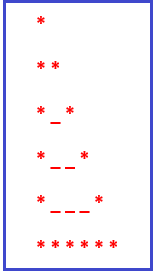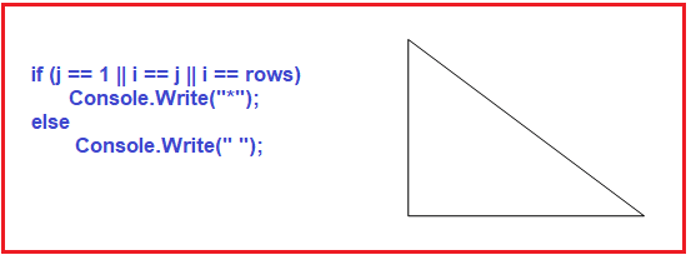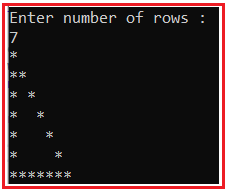# Hollow Right Triangle Star Pattern in C#

## Hollow Right Triangle Star Pattern in C# with Examples

In this article, I am going to discuss How to implement Hollow Right Triangle Star Pattern Program in C# with Examples. Please read our previous article where we How to implement the Downward/Reversed Mirrored Right Triangle Star Pattern Program in C#. Please have a look at the below image which shows the Hollow Right Triangle Star Pattern.##### Understanding the Hollow Right Triangle Star Pattern
1. In the first row, we have 1 star.
2. In the second row, we have 2 stars and 0 blank spaces in between stars.
3. In the third row, we have 2 stars and 1 blank space in between stars.
4. In the fourth row, we have 2 stars and 2 blank spaces in between stars.
5. In the fifth row, we have 3 stars and 2 blank spaces in between stars.
6. In the sixth row (the last row), we have 6(equal to row) stars.From the above pattern, it is clear that at each row (starting from the 2nd row) the number of blank spaces is increasing +1 till (row-1).

##### How to Implement the Right Triangle Star Pattern in C#?

To solve the above pattern,

1. We are going to use two for loops. The outer for loop and the inner for loop.
2. The outer for loop will be used to handle the rows one by one and the inner for loop will be used to handle the columns one by one.
3. The inner for loop will be used to print the stars and blank spaces according to row and the outer for loop job is to go on a new line after printing the current row stars and blank spaces.
4. The right Triangle has three parts the height, breadth, and hypotenuse
5. In the inner for loop, we have an if condition as shown below6. j==1 will help to print the height stars.
7. i==j will help to print the hypotenuse stars.
8. i==rows will help to print the breadth stars.

##### C# Program to Print Hollow Right Angle Triangle Star Pattern

The following C# Program will allow the user to input the number of rows and then print Hollow Right Angle Triangle Star Pattern on the console.

```using System;
public class HollowRightTriangleStarPattern
{
public static void Main()
{
Console.WriteLine("Enter number of rows :");
for (int i = 1; i <= rows; i++)
{
for (int j = 1; j <= i; j++)
{
if (j == 1 || i == j || i == rows)
Console.Write("*");
else
Console.Write(" ");
}
Console.WriteLine();
}